MORE IN Analog Electronics - 1
MU Electronics and Telecom Engineering (Semester 3)
Analog Electronics - 1
May 2011
Total marks: --
Total time: --
INSTRUCTIONS
(1) Assume appropriate data and state your reasons
(2) Marks are given to the right of every question
(3) Draw neat diagrams wherever necessary

1 A full Wave rectifier using a centre tapped transformer with two diodes gives output voltage of 250 V to a resistive load, the current being 75±25mA. If the ripple factor is 0.001, calculate the specification of the devices and components required if the filter used are-
(i) L section (LC) filter, (ii) ?- Filter and (iii) C filter. Draw complete circuit diagrams each case
20 M

2 (a) Design a Single stage RC coupled amplifier using FET BFW-11 biased to provide 3.5mA to achieve voltage gain of 10, lower cut of frequency of 20 Hz and to provide Vo=2.5V.
15 M
2 (b) For the designed amplifier find Av, Ri, Ro
5 M

3 (a) Determine the values of big components for CE configuration if VCC =12V,VCE=6V, RC=1K, VBE=0.6 V, hFE=180 for the following circuits -
1. Fixed bias without RE
2. Voltage divider biasing with VRE=1 V
10 M
3 (b) Determine Q Point and draw the dc load line for the following circuit.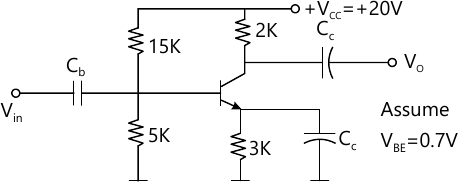10 M

4 (a) For the following circuit, determine Av, Ri, Ro.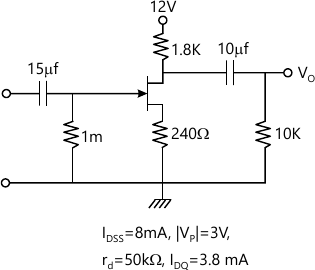10 M
4 (b) For a CE amplifier derive the expressions for Av, AI, Zi and Zo.
10 M

5 (a) For the following circuit determine d.c. output voltage, ripple factor and ripple at the output Assume diodes to be ideal.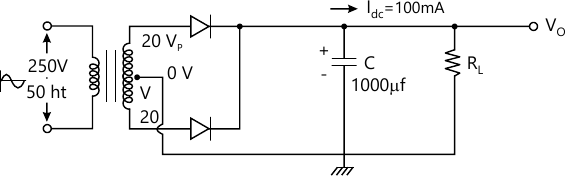10 M
5 (b) What are the causes of instability of operating point of a BJT amplifier? Derive the expression for stability factor SICO for voltage divider bias circuit.
10 M

6 (a) For the amplifier shown in following figure, determine- 1. Gm, rd 2. Zi, Zo, Av values in each case for both with and without rd.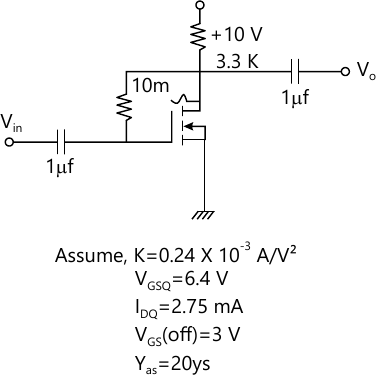10 M
6 (b) For the following circuit, determine IDQ and verify if the FET will operate in punch-off region.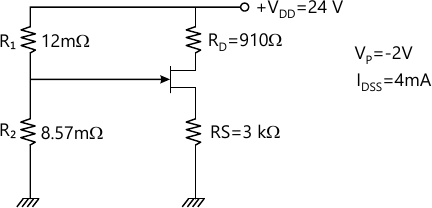10 M

7 (a) Power Mosfet-Characteristics, ratings and application.
7 M
7 (b) Schottkey diode-characteristics, ratings and application.
7 M
7 (c) UJT- Characteristics, rating and application.
7 M
7 (d) IGBT-Characteristics, rating and application.
7 M

More question papers from Analog Electronics - 1